Call 761-5001                Now Accepting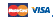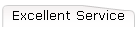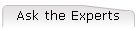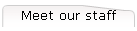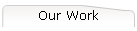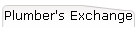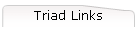Pay Invoices We now accept credit cards for quick easy payments.Call Mike at 761-5001 to make a payment.
 Ask the Experts It is a good idea to remove all hoses from exterior faucets during the winter.  >>Read More.

Thanks,    Ricky.

This page contains answers to common questions handled by our service staff, along with some tips and tricks that we have found useful.

#### Always remove hoses from your house in the Winter.

It is a good idea to remove all hoses from exterior faucets during the winter.  Hoses which are attached can still have water in them which will freeze and expand sometimes causing your faucet to crack.  It is also a good idea to replace old faucets with 'Freeze Proof' faucets which cut off the water ten to twelve inches inside the wall where it is warmer.  This helps reduce the risk of your faucets freezing and cracking.

#### Where can I find ... ?

This is the answer to the question. This is the answer to the question. This is the answer to the question. This is the answer to the question. This is the answer to the question. This is the answer to the question. This is the answer to the question. This is the answer to the question.

#### Why doesn't ... ?

This is the answer to the question. This is the answer to the question. This is the answer to the question. This is the answer to the question. This is the answer to the question. This is the answer to the question. This is the answer to the question. This is the answer to the question.

#### Who is ... ?

This is the answer to the question. This is the answer to the question. This is the answer to the question. This is the answer to the question. This is the answer to the question. This is the answer to the question. This is the answer to the question. This is the answer to the question.

#### What is ... ?

This is the answer to the question. This is the answer to the question. This is the answer to the question. This is the answer to the question. This is the answer to the question. This is the answer to the question. This is the answer to the question. This is the answer to the question.

This is the answer to the question. This is the answer to the question. This is the answer to the question. This is the answer to the question. This is the answer to the question.

#### When is ... ?

This is the answer to the question. This is the answer to the question. This is the answer to the question. This is the answer to the question. This is the answer to the question. This is the answer to the question. This is the answer to the question. This is the answer to the question.

This is the answer to the question. This is the answer to the question. This is the answer to the question. This is the answer to the question. This is the answer to the question.